# Two ages

Gabrielle is 9 years younger than Mikhail. The sum of their ages is 87. What is Mikhail's age?

m =  48

### Step-by-step explanation:

m+(m-9)=87

2m = 96

m = 48

Our simple equation calculator calculates it.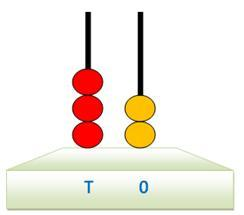We will be pleased if You send us any improvements to this math problem. Thank you!Tips to related online calculators
Do you have a linear equation or system of equations and looking for its solution? Or do you have a quadratic equation?
Do you want to convert time units like minutes to seconds?

## Related math problems and questions:

• The agesThe ages of the four sons make an arithmetic sequence, the sum of which is the age of the father today. In three years, the father's age will be the sum of the ages of the three eldest sons, and in the next two years and three months, the father's age wil
• TroopsThere are two leaders in the tourist group, with an average age of 30 years and several children with an average age of 10 years. The total age of the group is 12 years. How many children are in the group?
• Ages 2A man's age is 4 times his son's age. After 5 years he will be just twice his son's age, find their ages.
• CarlaCarla is 5 years old, and Jim is 13 years younger than Peter. One year ago, Peter's age was twice the sum of Carla's and Jim's age. Find the present age of each one of them.
• Age problemsA) Alex is 3 times as old as he was 2 years ago. How old is he now? b) Casey was twice as old as his sister 3 years ago. Now he is 5 years older than his sister. How old is Casey? c) Jessica is 4 years younger than Jennifer now. In 10 years, Jessica will
• Three men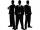Alex is half younger than Jan, which is one-third younger than George. The sum of their ages is 48. How are these three men old?
• Large familyThe average age of all family members (children, mother, father, grandmother, grandfather) is 29 years. The average age of parents is 40 years, grandparents 66 years and all children are 5 years. How many children are there in this family?
• AgesFather is 36 years old, and his daughter is 4 years old. Write down the ratio of the age of father and daughter. In what ratio will the ages of father and daughter after 4 years?
• Daughters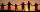The man conducting the census asks a woman to age of three daughters. Woman says when multiply the age getnumber 72; if their ages add up, get a number of our house, as you see. The man says: That is not enough to calculate their ages. She says: my oldest
• Three siblings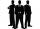Three siblings have birthday in one day-today. Together they have 35 years today. The youngest is three years younger than middle and the oldest is 5 years older than middle. How old is each?
• Family and age ratiosThe age of father and son are in the ratio 10:3; the age of father and daughter are in the ratio 5:2. How old are father and son if the daughter is 20 years old? In what ratio is age sister and brother?
• Age questionJano is three times older than Zdeno. Zdeno is 12 years younger than Janko. How old is Jano, and how old is Zdeno?
• SuzanSusan's age will be after 12 years four times as much as twelve years ago. How old is Susan now?
• GranddaughterIn 2014, the sum of the ages of Meghan's aunt, her daughter and her granddaughter was equal to 100 years. In what year was the granddaughter born, if we know that the age of each can be expressed as the power of two?
• Digits of age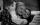The product of the digits of Andrew age as 6 years ago and not equal to 0. Andrew's age is also the youngest possible age with these two conditions. After how many years will the product of the digits of Andrew age again the same as today?
• Mary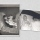Mary is 12 years old, which is 40% of the age of her mother. How many percent of her mother's age will Mary have after the next 12 years?
• 45th birthdayThis year Mrs. Clever celebrated her 45th birthday. Her three children are now 7, 11 and 15 years old. In how many years will Mrs. Clever's age be equal to the sum of her children's years?